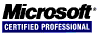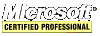## Insertion Sort Algorithm in C/C++

Insertion sort is the fastest of the O(n2) algorithms. It's an impressive little algorithm for sorting small arrays. Use it to sort the smaller subarrays in quick sort giving approximately a 20% performance increase.

See the Algorithm Study for more details.

`````` // insertion sort algorithm
void insertion_sort(int array[], const unsigned int size) {
for (unsigned int i = 1; i < size; i++) {
int temp = array[i];
unsigned int j = i;
while (j > 0 && temp < array[j - 1]) {
array[j] = array[j - 1];
j--;
}
array[j] = temp;
}
}
``````